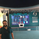8398 views
8398
Credits inline. Enjoy :)
```//
// @author LazyBear
// @credits http://freethinkscript.blogspot.com/2009/05/only-scalpers-channel-that-you-will.html
//
study(title = "Scalper's Channel [LazyBear]", shorttitle="weak_volume_dependency [LB]", overlay=true)
length = input(20)
factor = input(15)
pi = atan(1)*4
Average(x,y) => (sum(x,y) / y)
scalper_line= plot(Average(close, factor) - log(pi * (atr(factor))), color=blue, linewidth=3)
hi = plot (highest(length), color=fuchsia)
lo = plot (lowest(length), color=fuchsia)```

## Abprallerthank you sir.It will gratefully accepted if any possible strategy example for this. Below one is reference but needs your touch to make it works very well. Main problem in strategy library is if i'm going to use sma(close,1) instead of "high" or "lower" it will not enter on current bar, it's working for next bar rather then the current bar while backtesting. This is common issue.. How do you deal with that ?

hi1=(highest(length))
lo1=(lowest(length))

short1=cross(high,hi1)
long1=cross(low,lo1)
if (long1)
strategy.entry(id = "Long", long = true, when = long1)
strategy.exit("Long TP", from_entry = "Long",profit =250,stop=250)
if (short1)
strategy.entry(id = "Short", long = false, when = short1)
strategy.exit("Short TP", from_entry = "Short",profit =250,stop=250)
Antworteni am new to all of this. I am struggling. How does one use this indicator on a different chart?
Antworten